# Examples

#### 2021-04-09

In this vignette we discuss some properties of a robust backfitting estimator for additive models, and illustrate the use of the package RBF that implements it. These estimators were originally proposed in Boente G, Martinez A, Salibian-Barrera M. (2017). See also Martinez A. and Salibian-Barrera M. (2021).

Below we analyze two data sets. The first one shows the robustness of the robust backfitting estimators when a small proportion of very large outliers are present in the training set, and compares them with those obtained with the standard backfitting algorithm. With the second example, we illustrate how these robust estimators can be interpreted as automatically detecting and downweighting potential outliers in the training set. We also compare the prediction accuracy of the robust and classical backfitting algorithms.

## Boston example

Consider the well-known Boston house price data of Harrinson and Rubinfeld (1978). This dataset was used as an example to model an additive model by Härdle et a. (2004). The data are available in the MASS package. It contains $$n=506$$ observations and 14 variables measured on the census districts of the Boston metropolitan area. Following the analysis in Härdle et a. (2004) we use the following 10 explanatory variables:

• crim: per capita crime rate by town ($$X_1$$),
• indus: proportion of non-retail business acres per town ($$X_2$$),
• nox: nitric oxides concentration (parts per 10 million) ($$X_3$$),
• rm: average number of rooms per dwelling ($$X_4$$),
• age: proportion of owner-occupied units built prior to 1940 ($$X_5$$),
• dis: weighted distances to five Boston employment centers ($$X_6$$),
• tax: full-value property tax rate per 10,000 ($$X_7$$),
• ptratio: pupil-teacher ratio by town ($$X_8$$),
• black: $$1,000(Bk-0.63)^2$$ where $$Bk$$ is the proportion of people of Afrom American descent by town ($$X_9$$),
• lstat: percent lower status of population ($$X_{10}$$).

The response variable $$Y$$ is medv, the median value of the owner-occupied homes in 1,000 USD, and the proposed additive model is $Y= \mu+ \sum_{j=1}^{10} g_j(\log(X_j))+ \epsilon.$ where $$\mu \in \mathbb{R}$$, $$g_j$$ are unknown smooth functions, and $$\epsilon$$ are random errors.

First we load the data, and transform the explanatory variables as required by the model above:

data(Boston, package='MASS')
dd <- Boston[, c(1, 3, 5:8, 10:14)]
dd[, names(dd) != 'medv'] <- log( dd[, names(dd) != 'medv'] )

Next, we load the RBF package

library(RBF)

The robust backfitting estimators for each additive component are computed using robust kernel-based local polynomial regression. The model to be fit is specified using the standard formula notation in R. We also need to specify the following arguments:

• windows: the bandwidths for the kernel estimators,
• degree: the degree of the polynomial used for the kernel local regression, defaults to 0,
• type: specifies the robust loss function, options are Huber or Tukey, defaults to Huber.

As with all kernel-based estimators, bandwidth selection is an important step. In this example we follow Härdle et al. (2004) and select bandwidths $$h_j$$, $$1 \le j \le 10$$, proportional to the standard deviation of the corresponding explanatory variables $$\log(X_j)$$. Specifically we set $$h_j = \hat{\sigma}_j / 2$$:

bandw <- apply(dd[, names(dd) != 'medv'], 2, sd) / 2

We are now ready to compute the robust backfitting estimators:

robust.fit <- backf.rob(medv ~ ., data = dd, degree = 0, type = 'Huber',
windows = bandw)

Information about the fit can be obtained using the summary method:

summary(robust.fit)
#>
#> Call:
#> backf.rob(formula = medv ~ ., data = dd, windows = bandw, degree = 0,
#>     type = "Huber")
#>
#> Estimate of the intercept:  22.20546
#> Estimate of the residual standard error:  0.22239
#> Robust multiple R-squared:  0.69872
#> Residuals:
#>  Min. 1st Qu. Median Mean 3rd Qu. Max.
#>  -18.41161 -1.251476 0.002993124 0.3273419 1.457033 31.67697

Note that the summary output includes a robust version of the R-squared coefficient, which is computed as $R^2_{rob}=\frac{\sum_{i=1}^n \rho\left((Y_i-\widehat{a})/\widehat{\sigma}\right)-\sum_{i=1}^n \rho\left(R_i/\widehat{\sigma}\right)}{\sum_{i=1}^n \rho\left((Y_i-\widehat{a})/\widehat{\sigma}\right)} \, ,$ where $$\rho$$ is the loss function used by the M-kernel smoothers (as determined by the argument type in the call to backf.rob), and $$\widehat{a}$$ is a robust location estimator for a model without explanatory variables: $$Y=a+\epsilon$$.

The plot method can be used to visualize the estimated additive components $$\hat{g}_j$$, displayed over the corresponding partial residuals: $R_{ij}=Y_i-\hat{\mu}-\sum_{k\neq i}\hat{g}_k(X_{ik}) \, , \quad 1 \le i \le 506\, , \quad 1 \le j \le 10 \, .$

plot(robust.fit)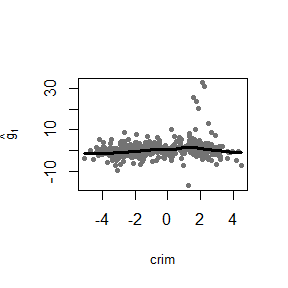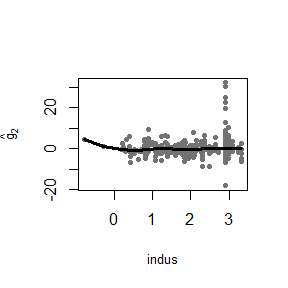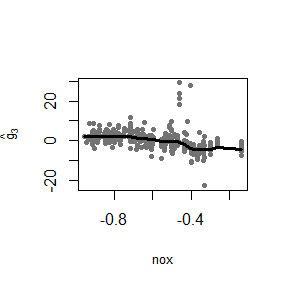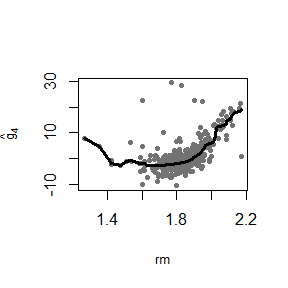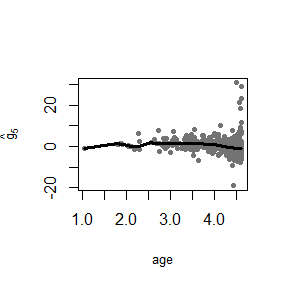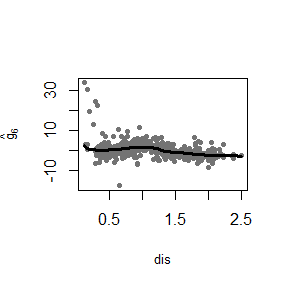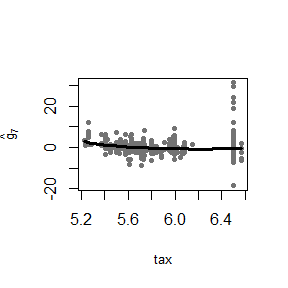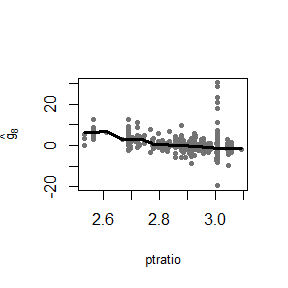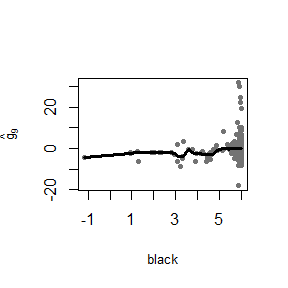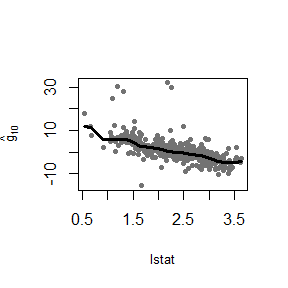By default, backf.rob computes fitted values on the training set. If predictions at a different specific point are desired, we can pass those points using the argumen point. For example, to obtain predicted values at a point po given by the average of the (log transformed) explanatory variables, we can use the following command (note that this step implies re-fitting the whole model):

po <- colMeans(dd[, names(dd) != 'medv'])
robust.fit1 <- backf.rob(medv ~ ., data = dd, degree = 0, type = 'Huber',
windows = bandw, point = po)

The values of the estimated components evaluated at the corresponding coordinates of po are returned in the $prediction element: robust.fit1$prediction
#>           crim      indus       nox        rm      age      dis        tax
#> [1,] 0.4502372 -0.2941408 0.5036051 -1.549345 0.484823 1.266611 -0.5900875
#>         ptratio     black      lstat
#> [1,] -0.2291248 0.1912795 -0.1634099

In order to illustrate the behaviour of the robust fit when outliers are present in the data, we artifically introduce 1% of atypical values in the response variable:

dd2 <- dd
dd2$medv[1:5]<- rep(400, 5) We now calculate the robust estimators using the contaminated data set. Note that we expect them to be very similar to those we obtained before with the original training set. robust.fit.new <- backf.rob(medv ~ ., data = dd2, degree = 0, type = 'Huber', windows = bandw, point = po) summary(robust.fit.new) #> #> Call: #> backf.rob(formula = medv ~ ., data = dd2, windows = bandw, point = po, #> degree = 0, type = "Huber") #> #> Estimate of the intercept: 22.21968 #> Estimate of the residual standard error: 0.22239 #> Robust multiple R-squared: 0.44603 #> Residuals: #> Min. 1st Qu. Median Mean 3rd Qu. Max. #> -18.35562 -1.249837 0.002044039 3.969449 1.489455 378.8072 robust.fit.new$prediction
#>           crim      indus       nox        rm       age      dis        tax
#> [1,] 0.3669282 -0.3464365 0.5552477 -1.573039 0.5296952 1.248268 -0.5550474
#>         ptratio     black      lstat
#> [1,] -0.2196106 0.1936705 -0.1590413

From the output above we verify that the predictions at the point po with both fits are very similar to each other.
Because the magnitude of the outliers affects the scale of the partial residuals plots, to compare both fits below we plot the robust estimators for each additive component trained on the original and contaminated data sets (without including the partial residuals). In green and dashed lines the robust estimator computed with the original data set, and in blue and solid lines the robust estimator computed with the contaminated data set. We see that, indeed, both sets of estimated additive components are very similar to each other.

for(j in 1:10) {
name.x <- names(dd)[j]
name.y <- bquote(paste(hat('g')[.(j)]))
oo <- order(dd2[,j])
plot(dd2[oo,j], robust.fit.new$g.matrix[oo,j], type="l", lwd=2, col='blue', lty=1, xlab=name.x, ylab=name.y) lines(dd2[oo,j], robust.fit$g.matrix[oo,j], lwd=2, col='green', lty=2)
}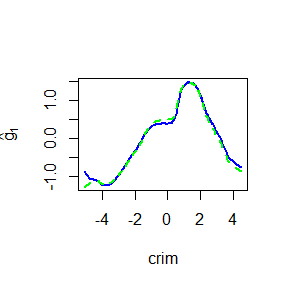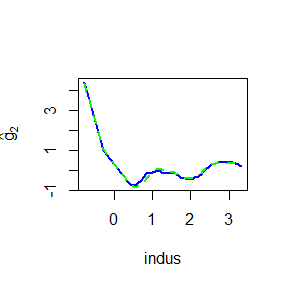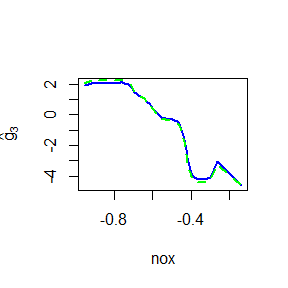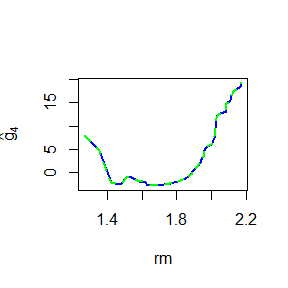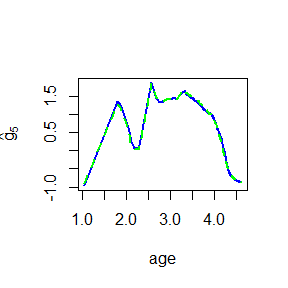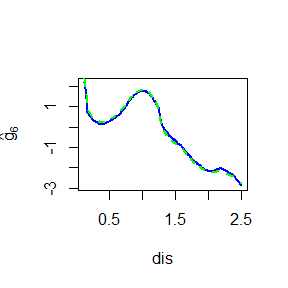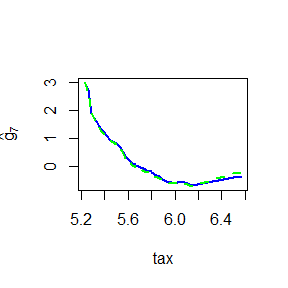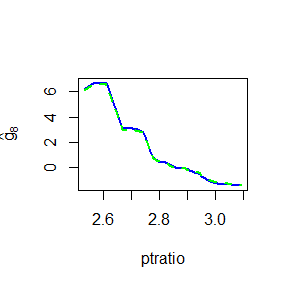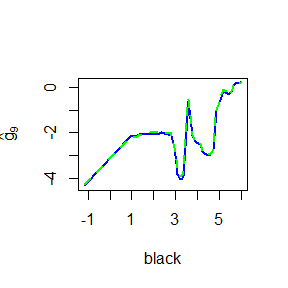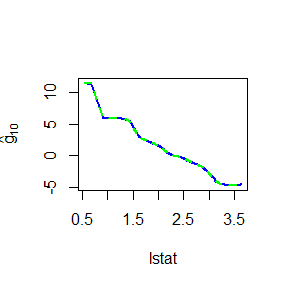It is easy to see that when we use the classical backfitting estimator the fits obtained when the training set contains outliers are dramatically different from the ones obtained with the original data set. We use the package gam to compute the standard backfitting algorithm (based on local kernel regression smoothers). The bandwidths used to compute the classical estimates are again proportional to the standard deviations of the explanatory variables, but we use a slightly larger coefficient to avoid numerical issues with the local fits. We set $$h_j=(3/4) \, \widehat{\sigma}_j$$, for $$1 \le j \le 10$$:

library(gam)
fit.gam <- gam(medv ~ lo(crim, span=1.62) + lo(indus, span=0.58) +
lo(nox, span=0.15) + lo(rm, span=0.08) +
lo(age, span=0.46) + lo(dis, span=0.40) +
lo(tax, span=0.30) + lo(ptratio, span=0.09) +
lo(black, span=0.58) + lo(lstat, span=0.45), data=dd)
fits <- predict(fit.gam, type='terms')
fit.gam.new <- gam(medv ~ lo(crim, span=1.62) + lo(indus, span=0.58) +
lo(nox, span=0.15) + lo(rm, span=0.08) +
lo(age, span=0.46) + lo(dis, span=0.40) +
lo(tax, span=0.30) + lo(ptratio, span=0.09) +
lo(black, span=0.58) + lo(lstat, span=0.45), data=dd2)
fits.new <- predict(fit.gam.new, type='terms')

In the plots below, the standard backfitting estimates calculated with the original Boston data set are shown with orange and dashed lines, while those obtained with the contaminated training set are shown with purple and solid lines.

for(j in 1:10) {
oo <- order(dd2[,j])
name.x <- names(dd)[j]
name.y <- bquote(paste(hat('g')[.(j)]))
plot(dd2[oo,j], fits.new[oo,j], type="l", lwd=2, col='purple', lty=1,
xlab=name.x, ylab=name.y)
lines(dd2[oo,j], fits[oo,j], lwd=2, col='darkorange2', lty=2)
}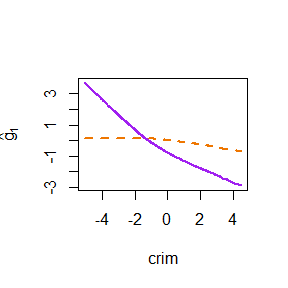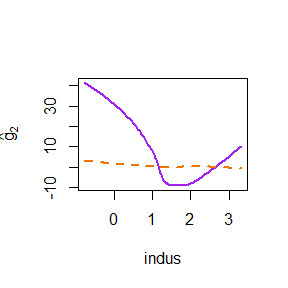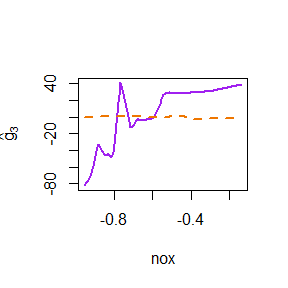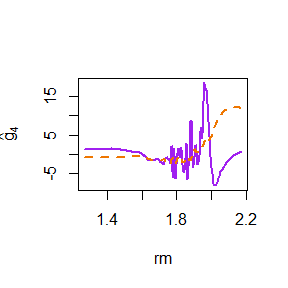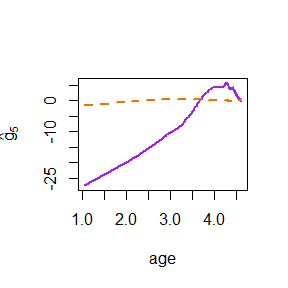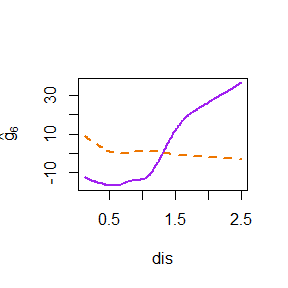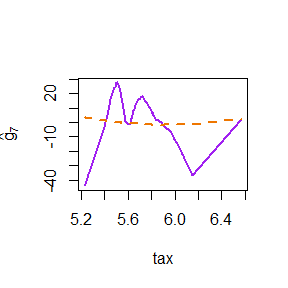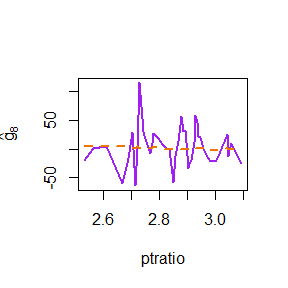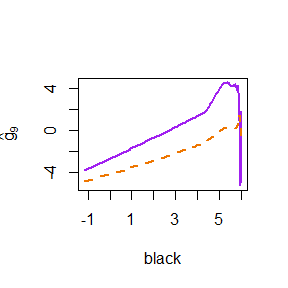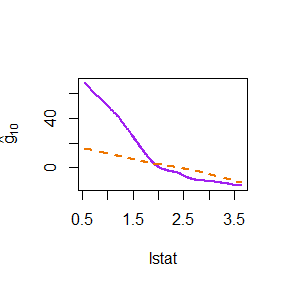## Airquality example

The airquality data set contains 153 daily air quality measurements in the New York region between May and September, 1973 (Chambers et al., 1983). The interest is in modeling the mean Ozone ($$\mbox{O}_3$$) concentration as a function of 3 potential explanatory variables: solar radiance in the frequency band 4000-7700 (Solar.R), wind speed (Wind) and temperature (Temp). We focus on the 111 complete entries in the data set.

data(airquality)
ccs <- complete.cases(airquality)
aircomplete <- airquality[ccs, c('Ozone', 'Solar.R', 'Wind', 'Temp')]
pairs(aircomplete[, c('Ozone', 'Solar.R', 'Wind', 'Temp')], pch=19, col='gray30')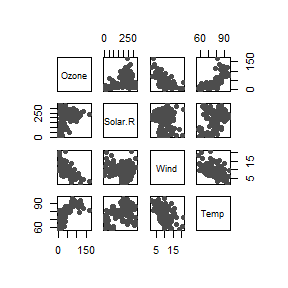The scatter plot suggests that the relationship between ozone and the other variables in not linear and so we propose using an additive regression model of the form $\begin{equation} \label{eq:ozone-model} \mbox{Ozone}=\mu+g_{1}(\mbox{Solar.R})+g_{2}(\mbox{Wind})+g_{3}(\mbox{Temp}) + \varepsilon \, . \end{equation}$ To fit this model above we use robust local linear kernel M-estimators and Tukey’s bisquare loss function. These choices are set using the arguments degree = 1 and type='Tukey' in the call to the function backf.rob. The model is specified with the standard formula notation in R. The argument windows is a vector with the bandwidths to be used with each kernel smoother. To estimate optimal values we used a robust leave-one-out cross-validation approach (see Boente et al., 2017). As a robust prediction error measure we use mu^2 + sigma^2 where mu and sigma are M-estimators of location and scale of the prediction errors, respectively.

library(RBF)

# Bandwidth selection with leave-one-out cross-validation
## Without outliers
# This takes a long time to compute (approx 380 minutes running
# R 3.6.1 on an Intel(R) Core(TM) i7-4790 CPU @ 3.60GHz)
a <- c(1/2, 1, 1.5, 2, 2.5, 3)
h1 <- a * sd(aircomplete[,2])
h2 <- a * sd(aircomplete[,3])
h3 <- a * sd(aircomplete[,4])
hh <- expand.grid(h1, h2, h3)
nh <- nrow(hh)
rmspe <- rep(NA, nh)
jbest <- 0
cvbest <- +Inf
n <- nrow(aircomplete)
for(i in 1:nh) {
# leave-one-out CV loop
preds <- rep(NA, n)
for(j in 1:n) {
tmp <- try( backf.rob(Ozone ~ Solar.R + Wind + Temp, point = aircomplete[j, -1],
windows = hh[i, ], epsilon = 1e-6, data = aircomplete,
degree = 1, type = 'Tukey', subset = c(-j) ))
if (class(tmp) != "try-error") {
preds[j] <- rowSums(tmp$prediction) + tmp$alpha
}
}
tmp.re <- RobStatTM::locScaleM(preds - aircomplete$Ozone, na.rm=TRUE) rmspe[i] <- tmp.re$mu^2 + tmp.re$disper^2 if( rmspe[i] < cvbest ) { jbest <- i cvbest <- rmspe[i] } } (bandw <- hh[jbest,]) The resulting bandwidths are: bandw <- c(136.7285, 10.67314, 4.764985) Now we use the robust backfitting algorithm to fit an additive model using Tukey’s bisquare loss (the default tuning constant for this loss function is 4.685) and the optimal bandwidths. fit.full <- backf.rob(Ozone ~ Solar.R + Wind + Temp, windows = bandw, epsilon = 1e-6, degree = 1, type = 'Tukey', subset = ccs, data = airquality) We can visually explore the estimated additive functions plotted over the corresponding partial residuals using the method plot: plot(fit.full)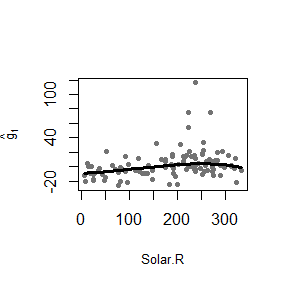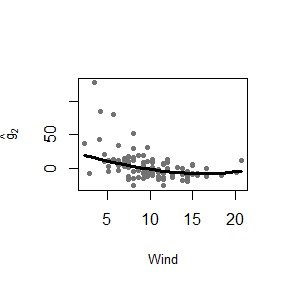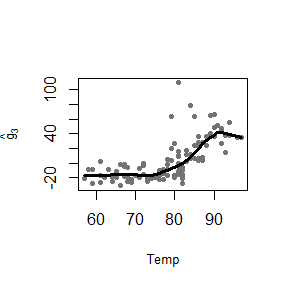As before, we use the R package gam to compute the classical additive model estimators for this model. Optimal bandwidths were calculated using leave-one-out cross-validation as before: library(gam) a <- c(.3, .4, .5, .6, .7, .8, .9) hh <- expand.grid(a, a, a) nh <- nrow(hh) jbest <- 0 cvbest <- +Inf n <- nrow(aircomplete) for(i in 1:nh) { fi <- rep(0, n) for(j in 1:n) { tmp <- gam(Ozone ~ lo(Solar.R, span=hh[i,1]) + lo(Wind, span=hh[i,2]) + lo(Temp, span=hh[i,3]), data = aircomplete, subset=c(-j)) fi[j] <- as.numeric(predict(tmp, newdata=aircomplete[j, -1], type='response')) } ss <- mean((aircomplete$Ozone - fi)^2)
if(ss < cvbest) {
jbest <- i
cvbest <- ss
}
}
(hh[jbest,])
# Var1 Var2 Var3
# 0.7  0.7  0.5

The optimal bandwidths are 0.7, 0.7 and 0.5 for Solar.R, Wind and Temp, respectively, and we use them to compute the backfitting estimators:

fit.gam <- gam(Ozone ~ lo(Solar.R, span=.7) + lo(Wind, span=.7)+
lo(Temp, span=.5), data = aircomplete)

Both classical (in magenta and dashed lines) and robust (in blue and solid lines) fits are shown in the following plot together with the partial residuals obtained by the robust fit.

x <- as.matrix( aircomplete[ , c('Solar.R', 'Wind', 'Temp')] )
y <- as.vector( aircomplete[ , 'Ozone'] )
fits <- predict(fit.gam, type='terms')
for(j in 1:3) {
re <- fit.full$yp - fit.full$alpha - rowSums(fit.full$g.matrix[,-j]) plot(re ~ x[,j], type='p', pch=20, col='gray45', xlab=colnames(x)[j], ylab='') oo <- order(x[,j]) lines(x[oo,j], fit.full$g.matrix[oo,j], lwd=2, col='blue', lty=1)
lines(x[oo,j], fits[oo,j], lwd=2, col='magenta', lty=2)
}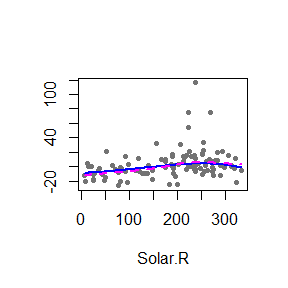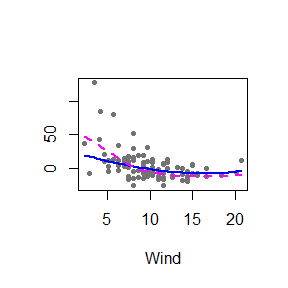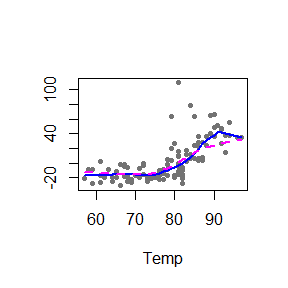The two fits differ mainly on the estimated effects of wind speed and temperature. The classical estimate for $$g_1(\mbox{Temp})$$ is consistently lower than the robust counterpart for $$\mbox{Temp} \ge 85$$. For wind speed, the non-robust estimate $$\hat{g}_2(\mbox{Wind})$$ suggests a higher effect over Ozone concentrations for low wind speeds than the one given by the robust estimate, and the opposite difference for higher speeds.

Since residuals from a robust fit can generally be used to detect the presence of atypical observations in the training data, we plot the boxplot of the residuals obtained by the robust fit and 4 possible outlying points (indicated with red circles) can be observed.

re.ro <- residuals(fit.full)
ou.ro <- boxplot(re.ro, col='gray80')$out in.ro <- (1:length(re.ro))[ re.ro %in% ou.ro ] points(rep(1, length(in.ro)), re.ro[in.ro], pch=20, col='red')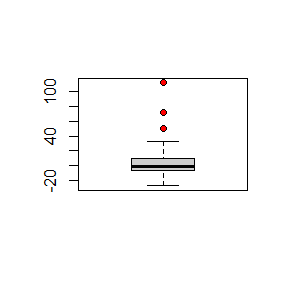(in.ro) #>  23 34 53 77 We highlight these suspicious observations on the scatter plot. cs <- rep('gray30', nrow(aircomplete)) cs[in.ro] <- 'red' os <- 1:nrow(aircomplete) os2 <- c(os[-in.ro], os[in.ro]) pairs(aircomplete[os2, c('Ozone', 'Solar.R', 'Wind', 'Temp')], pch=19, col=cs[os2])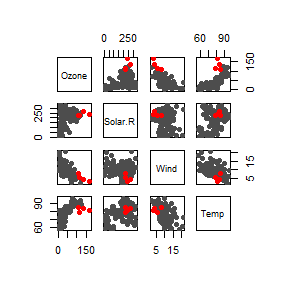Note that not all these suspected atypical observations are particularly extreme, or directly evident on the scatter plot. However, as we will show below, they do have an important effect on the estimates of the components of the additive model. The partial residuals corresponding to these points can be also visualized in red in the plot of the estimated curves. # Plot both fits (robust and classical) x <- as.matrix( aircomplete[ , c('Solar.R', 'Wind', 'Temp')] ) y <- as.vector( aircomplete[ , 'Ozone'] ) fits <- predict(fit.gam, type='terms') for(j in 1:3) { re <- fit.full$yp - fit.full$alpha - rowSums(fit.full$g.matrix[,-j])
plot(re ~ x[,j], type='p', pch=20, col='gray45', xlab=colnames(x)[j], ylab='')
points(re[in.ro] ~ x[in.ro,j], pch=20, col='red')
oo <- order(x[,j])
lines(x[oo,j], fit.full$g.matrix[oo,j], lwd=2, col='blue', lty=1) lines(x[oo,j], fits[oo,j], lwd=2, col='magenta', lty=2) }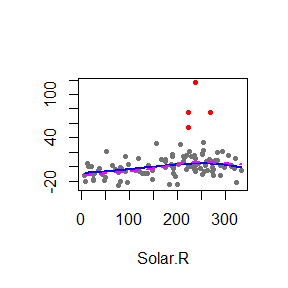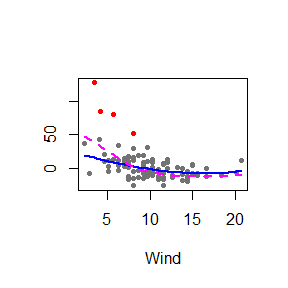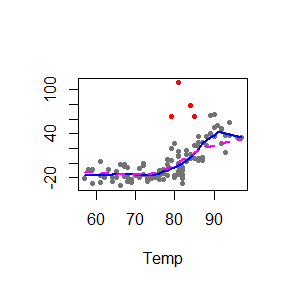To investigate whether the differences between the robust and non-robust estimators are due to the outliers, we recomputed the classical fit after removing them. We ran a similar leave-one-out cross-validation experiment to select the spans for each the 3 univariate smoothers. airclean <- aircomplete[-in.ro, c('Ozone', 'Solar.R', 'Wind', 'Temp')] a <- c(.3, .4, .5, .6, .7, .8, .9) hh <- expand.grid(a, a, a) nh <- nrow(hh) jbest <- 0 cvbest <- +Inf n <- nrow(airclean) for(i in 1:nh) { fi <- rep(0, n) for(j in 1:n) { tmp <- gam(Ozone ~ lo(Solar.R, span=hh[i,1]) + lo(Wind, span=hh[i,2]) + lo(Temp, span=hh[i,3]), data=airclean, subset=c(-j)) fi[j] <- as.numeric(predict(tmp, newdata=airclean[j,], type='response')) } ss <- mean((airclean$Ozone - fi)^2)
if(ss < cvbest) {
jbest <- i
cvbest <- ss
}
}
(hh[jbest,])
# Var1 Var2 Var3
# 0.7  0.8  0.3

We use the optimal bandwidths to compute non-robust fit.

airclean <- aircomplete[-in.ro, c('Ozone', 'Solar.R', 'Wind', 'Temp')]
fit.gam2 <- gam(Ozone ~ lo(Solar.R, span=.7) + lo(Wind, span=.8)+
lo(Temp, span=.3), data=airclean) 

The following plot shows the estimated curves obtained with the classical estimator using the clean data together with the robust ones (computed on the whole data set). Outliers are highlighted in red.

fits2 <- predict(fit.gam2, type='terms')
dd2 <- aircomplete[-in.ro, c('Solar.R', 'Wind', 'Temp')]
for(j in 1:3) {
re <- fit.full$yp - fit.full$alpha - rowSums(fit.full$g.matrix[,-j]) plot(re ~ x[,j], type='p', pch=20, col='gray45', xlab=colnames(x)[j], ylab='') points(re[in.ro] ~ x[in.ro,j], pch=20, col='red') oo <- order(dd2[,j]) lines(dd2[oo,j], fits2[oo,j], lwd=2, col='magenta', lty=2) oo <- order(x[,j]) lines(x[oo,j], fit.full$g.matrix[oo,j], lwd=2, col='blue', lty=1)
}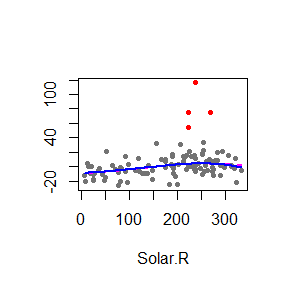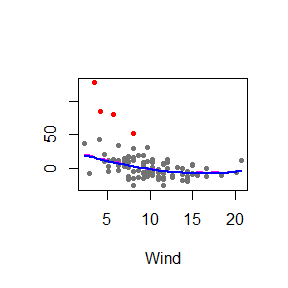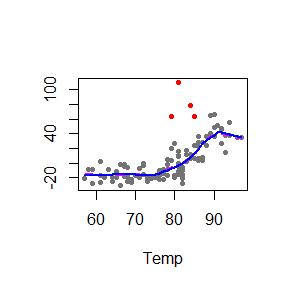Note that both fits are now very close. An intuitive interpretation is that the robust fit has automatically down-weighted potential outliers and produced estimates very similar to the classical ones applied to the clean observations.

### Prediction comparison

Finally, we compare the prediction accuracy obtained with each of the fits. Because we are not interested in predicting well any possible outliers in the data, we evaluate the quality of the predictions using a 5%-trimmed mean squared prediction error (effectively measuring the prediction accuracy on 95% of the data). We use this alpha-trimmed mean squared function:

tms <- function(a, alpha=.1) {
# alpha is the proportion to trim
a2 <- sort(a^2, na.last=NA)
n0 <- floor( length(a) * (1 - alpha) )
return( mean(a2[1:n0], na.rm=TRUE) )
}

We use 100 runs of 5-fold CV to compare the 5%-trimmed mean squared prediction error of the robust fit and the classical one. Note that the bandwidths are kept fixed at their optimal value estimated above.

dd <- airquality
dd <- dd[complete.cases(dd), c('Ozone', 'Solar.R', 'Wind', 'Temp')]
# 100 runs of K-fold CV
M <- 100
# 5-fold
K <- 5
n <- nrow(dd)
# store (trimmed) TMSPE for robust and gam, and also
tmspe.ro <- tmspe.gam <- vector('numeric', M)
set.seed(123)
ii <- (1:n)%%K + 1
for(runs in 1:M) {
tmpro <- tmpgam <- vector('numeric', n)
ii <- sample(ii)
for(j in 1:K) {
fit.full <- backf.rob(Ozone ~ Solar.R + Wind + Temp,
point=dd[ii==j, -1], windows = bandw,
epsilon = 1e-6, degree = 1, type = 'Tukey',
subset = (ii!=j), data = dd)
tmpro[ ii == j ] <- rowSums(fit.full$prediction) + fit.full$alpha
fit.gam <- gam(Ozone ~ lo(Solar.R, span=.7) + lo(Wind, span=.7)+
lo(Temp, span=.5), data = dd[ii!=j, ])
tmpgam[ ii == j ] <- predict(fit.gam, newdata=dd[ii==j, ], type='response')
}
tmspe.ro[runs] <- tms( dd$Ozone - tmpro, alpha=0.05) tmspe.gam[runs] <- tms( dd$Ozone - tmpgam, alpha=0.05)
}

These are the boxplots. We see that the robust fit consistently fits the vast majority (95%) of the data better than the classical one.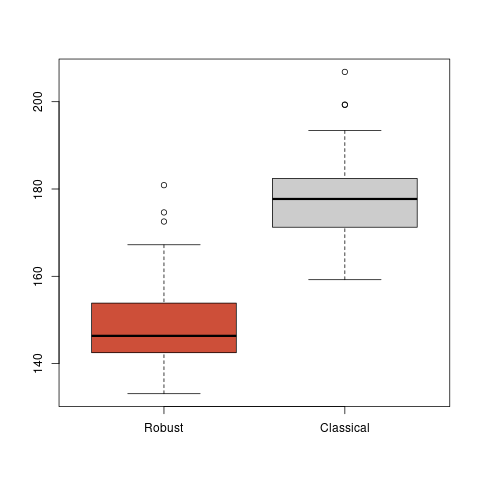Boxplots of the prediction errors

## Bibliography

Boente G, Martinez A, and Salibian-Barrera M. (2017) Robust estimators for additive models using backfitting. Journal of Nonparametric Statistics, 29, 744-767. DOI: 10.1080/10485252.2017.1369077

Chambers, J. M., Cleveland W. S., Kleiner B. and Tukey A. (1983). Graphical Methods for Data Analysis. 2nd edition. Chapman & Hall. DOI: 10.1201/9781351072304

Härdle, W., Müller, M., Sperlich, S. and Werwatz, A. (2004). Nonparametric and Semiparametric Models. Springer. DOI: 10.1007/978-3-642-17146-8

Harrinson, D. and Rubinfeld, D. L. (1978). Hedonic prices and the demand for clean air. J. Environ. Economics and Management, 5, 81-102. DOI: 10.1016/0095-0696(78)90006-2

Martinez A, and Salibian-Barrera M. (2021) RBF: An R package to compute a robust backfitting estimator for additive models. Journal of Open Source Software, 6(60). DOI: 10.21105/joss.02992